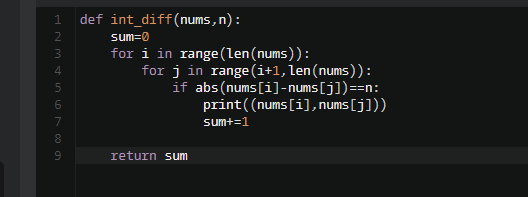# 【每日一题0906】数字间隔请编写一个函数，接收两个参数：一个是数字列表nums，一个是数字n。返回列表中任意两个数字的差值刚好是n的所有组合的个数。

``````def int_diff(nums: list, n: int) -> int:
pass

assert int_diff([1, 1, 5, 6, 9, 16, 27], 4) == 3
assert int_diff([1, 1, 3, 3], 2) == 4
``````

def int_diff(nums: list, n: int):
count=0
for i in range(0,len(nums)):
for j in range(0,len(nums)):
if nums[i]-nums[j]==n:
count+=1
print(count)
return count

@jisonglin 同学太优秀了～～

``````def int_diff(nums: list, n: int):
count=0
for i in range(0,len(nums)):
for j in range(0,len(nums)):
if nums[i]-nums[j]==n:
count += 1
return count
``````
``````def int_diff(nums: list, n: int) -> tuple:
count = 0
result = list()
for i in range(len(nums) - 1):
for j in range(i + 1, len(nums)):
# 计算绝对值
if abs(nums[i] - nums[j]) == n:
count += 1
result.append((nums[i], nums[j]))
return count, result
``````
``````def int_diff(nums: list, n: int) -> int:
# 定义匹配的组合个数
group_count = 0
# 第一层 for 循环
for i in range(len(nums)):
# 取第一个数
num1 = nums[i]
# 第二层 for 循环，以 i+1 开始
for j in range(i + 1, len(nums)):
# 取第二个数
num2 = nums[j]
# 判断两个数的差值是否为 n
if abs(num2 - num1) == n:
# 是则匹配的组合个数 +1
group_count = group_count + 1
# 返回匹配的组合个数
return group_count

assert int_diff([1, 1, 5, 6, 9, 16, 27], 4) == 3
assert int_diff([1, 1, 3, 3], 2) == 4
````````````   /**
* 请编写一个函数，接收两个参数：一个是数字列表nums，一个是数字n。返回列表中任意两个数字的差值刚好是n的所有组合的个数。
* 示例：
* 输入：(nums=[1, 1, 5, 6, 9, 16, 27], n=4)，输出3。因为组合情况可以是：(1,5), (1,5), (5,9)
* @param arrayList
* @param integer
* @return
*/
public Integer IntDiff(ArrayList<Integer> arrayList,Integer integer){
int result =0;
for (int i = 0; i < arrayList.size(); i++) {
for (int j = i; j <arrayList.size() ; j++) {
if (arrayList.get(j) - arrayList.get(i) == integer){
result++;
}
}
}
return result;
}

@Test
public void TestIntDiff(){
ArrayList<Integer> integers = new ArrayList<>();
assert IntDiff(integers, 4) == 3;
ArrayList<Integer> integers1 = new ArrayList<>();
assert IntDiff(integers1, 2) ==4;

}
``````

def int_diff(nums: list, n: int) → int:
list1=
a=len(nums)
for i in range(0,a):
for j in range(0,a):
if nums[j]-nums[i]==n:
list1.append((nums[i],nums[j]))
c=len(list1)
return c

### 参考题解

``````import itertools

def int_diff(nums: list, n: int) -> list:

pairs = list(itertools.combinations(nums, 2))
valid = [(x, y) for x, y in pairs if abs(x - y) == n]

return len(valid)

``````

1. 利用内置生成器函数combinations，得到nums任意2个元素配对的组合结果pairs
2. 遍历pairs，统计两个数的差值刚好是n的配对的个数